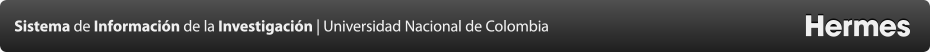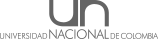Proyectos
3D numerical model in cylindrical coordinates to estimate the temperature during steam injection in heavy oil reservoirs
Resumen
The temperature is the most important variable during thermal recovery processes with steam injection, since increasing the temperature it is possible to reduce the high viscosity of fluid inside the heavy oil reservoirs. Some analytical and numerical models have been developed in order to represent the temperature behavior during steam injection but it is not common to find a model that been numerical, to 3 dimensions and presented in cylindrical coordinates. In this work a 3D temperature model is presented, this model is solved numerically in finite differences and developed cylindrical coordinates. The particularity of this model is that its numerical approach is reached from Lax Equivalence Theorem which guarantees the principles of consistency, stability, and convergence for numerical solution. Finally, a simple case of fluid injection at high temperature is modeled using a range of values that define different possibilities of numerical schemes, finding that an implicit scheme is the best option to solve the 3D diffusion-advection equation.
Convocatoria
 Nombre de la convocatoria: CONVOCATORIA DE APOYO PARA CORRECCIÓN DE ESTILO DE ARTÍCULOS DE INVESTIGACIÓN Modalidad: Modalidad única
Responsable